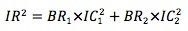### Why should I choose AnalystNotes?

AnalystNotes specializes in helping candidates pass. Period.

The fundamental law is additive in the square of information ratio.• Can be applied to different asset categories.
• Can be applied to one asset category + market timing.
• Can be applied to an international portfolio.
• It holds across managers, assuming the allocation across the managers is optimal.

#### Practice Question 1

Suppose the information ratio for a manager is 0.5. The manager decides to follow an additional 100 stocks with quarterly forecasts. The information coefficient for these forecasts is 0.02. What will be the manager's new information ratio?

A. 0.54.
B. 0.60.
C. 0.64.

IR2 = 0.52 + 100 x 4 x 0.022 = 0.41, IR = 0.64.

#### Practice Question 2

Suppose a manager follows 200 stocks and take a position on these stocks once a year. The manager's IC is 0.02. In the mean time the manager also makes a quarterly forecast on the market. His IC for market forecasts is 0.05. The manager's IR is:

A. 0.09.
B. 0.81.
C. 0.3.

IR2 = 200 x 0.022 + 4 x 0.052 = 0.09. IR = 0.3.

#### Practice Question 3

Suppose a pension fund hires 2 active managers with information ratios of 0.6 and 0.75, respectively. Assume the allocation across the managers is optimal. What is the information ratio of the pension fund?

A. 0.675.
B. 0.92.
C. 0.96.

IR2 = 0.62 + 0.752, IR = 0.96.

#### Practice Question 4

Suppose the information ratio for a manager is 0.5. The manager decides to follow an additional 25 stocks with semi-annual forecasts. The information coefficient for these forecasts is 0.04. What will be the manager's new information ratio?

A. 0.54.
B. 0.57.
C. 0.61.

IR2 = 0.52 + 25 x 2 x 0.042 = 0.33, IR = 0.57.

#### Practice Question 5

Suppose an insurance company hires 3 active managers with information ratios of 0.4, 0.5 and 0.6, respectively. Assume the allocation across the managers is optimal. What is the information ratio of the insurance company?

A. 0.5.
B. 0.77.
C. 0.88.